﻿ 固体火箭发动机装药动态燃速辨识方法
 舰船科学技术2020, Vol. 42Issue (2): 181-184PDF

1. 中国船舶重工集团公司第七一三研究所，河南 郑州 450015;
2. 河南省水下智能装备重点实验室，河南 郑州 450015

Dynamic burning rate identification of solid rocket motor propellant
LIU Zong-kui1,2, WANG Tai-kun1,2, WANG Hong-li1,2, LI Peng-yong1,2
1. The 713 Research Institute of CSIC, Zhengzhou 450015, China;
2. Henan Key Laboratory of Underwater Intelligence Equipment, Zhengzhou 450015, China
Abstract: Aiming at the common phenomenon that the static burning rate and the dynamic burning rate of modified double-base propellant for solid rocket motor were different. The main factors affecting the burning rate of solid propellant were analyzed. A method identifying the dynamic burning rate of solid rocket motor based on least square method is proposed. Comparisons between theoretical pressure curves calculated by identifying dynamic burning rate and test pressure curves, which show that this method improves the prediction accuracy of theoretical calculation model and has strong engineering application value.
Key words: burning rate identification     static burning rate     dynamic burning rate
0 引　言

1 燃速基本理论 1.1 燃速定义

 $\dot r = \frac{{{\rm d}e}}{{{\rm d}t}} {\text{。}}$
1.2 燃速定律

 $r = a{p^n} {\text{。}}$

1.3 影响推进剂燃速的因素

1）推进剂组分对燃速的影响

2）初温对燃速的影响

 ${\alpha _T} = {\left[ {\frac{1}{{\dot r}}\frac{{\partial \dot r}}{{\partial {T_i}}}} \right]_p} {\text{，}}$

 ${\alpha _T} = \frac{{{r_2} - {r_1}}}{{{r_1}({T_2} - {T_1})}} {\text{。}}$

3）密度对燃速的影响

4）压强对燃速的影响

5）燃速的加速度效应

2 静态燃速与动态燃速差异分析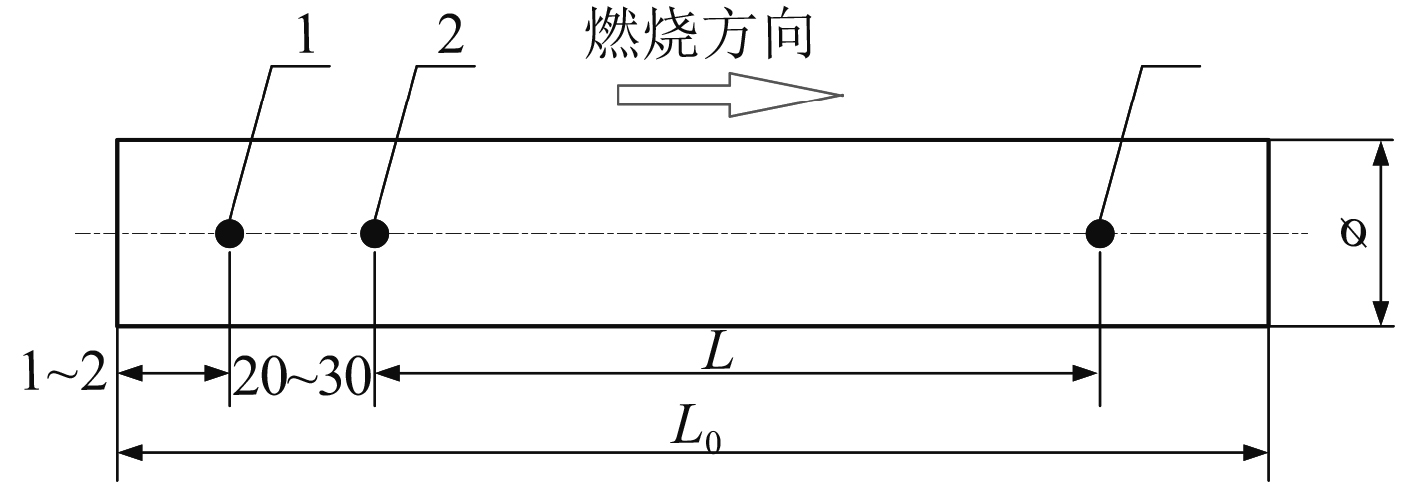图 1 燃速测试装药燃烧方向示意图（轴向燃烧） Fig. 1 Burning direction of test burning rate charge （axial burning）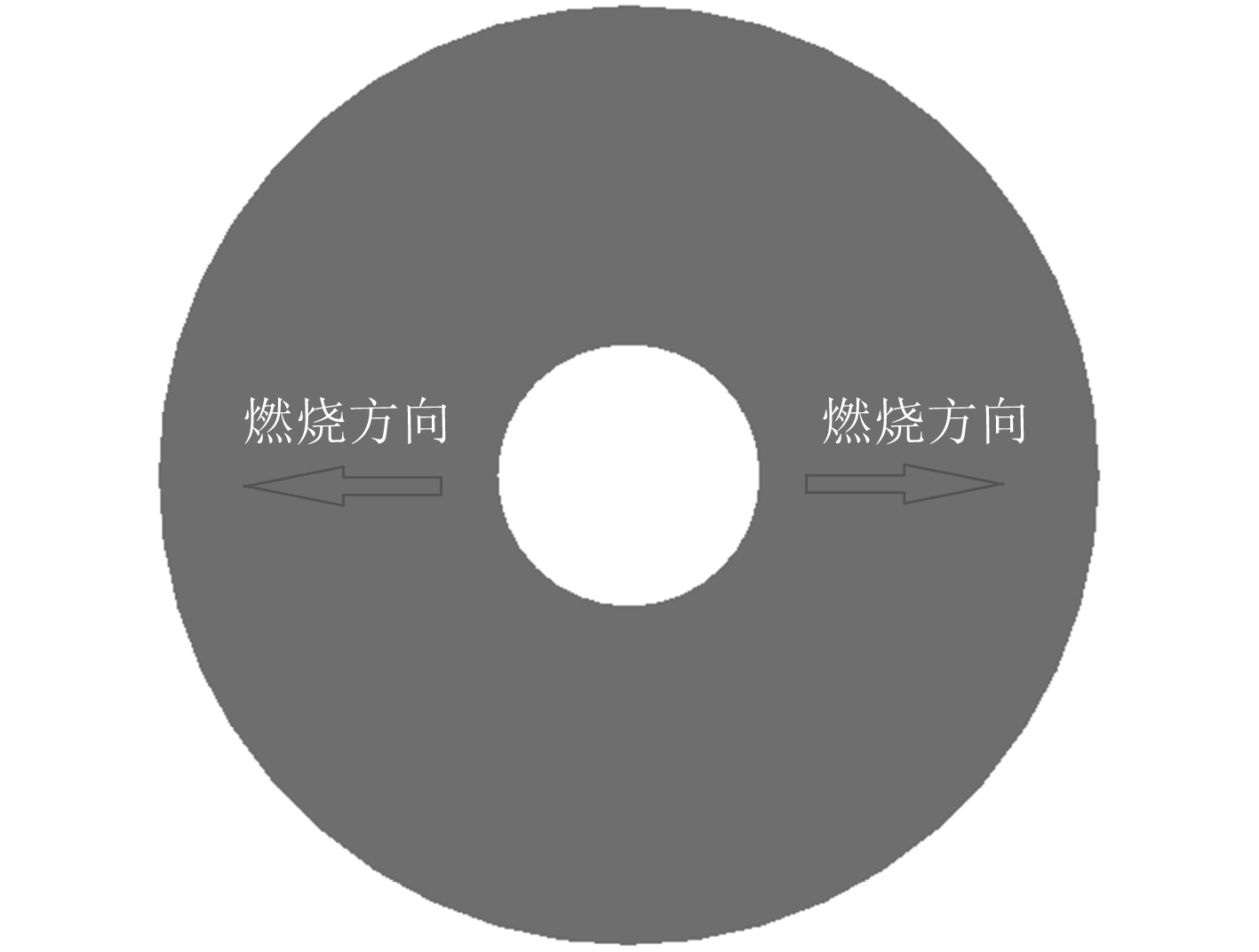图 2 装药实际燃烧方向示意图（径向燃烧） Fig. 2 Schematic diagram of actual combustion direction of charge （radial combustion）
3 燃速辨识理论基础 3.1 燃烧室头部平衡压强

 ${{t}} = \frac{{{{{V}}_{{\rm{c}}0}}}}{{(1 - {\rm{\nu }}){{k}}{{\left(\dfrac{2}{{{{k}} + 1}}\right)}^{\frac{{{{k}} + 1}}{{{{k}} - 1}}}}{{{C}}^*}{{At}}}}\ln \frac{{1 - {{\left(\dfrac{{{{{P}}_{{{ig}}}}}}{{{{{P}}_0}}}\right)}^{1 - {\rm{\nu }}}}}}{{1 - {{\left(\dfrac{{{{{P}}_{}}}}{{{{{P}}_0}}}\right)}^{1 - {\rm{\nu }}}}}} {\text{。}}$

 ${{{P}}_0} = {\left({{\rm{\rho }}_{{p}}}{{{U}}_1}{\rm{\varepsilon }}{{{C}}^*}\frac{{{{{A}}_{{b}}}}}{{{\rm{\mu }}{{\rm{\sigma }}_{{f}}}{{{A}}_{{t}}}}}\right)^{\frac{1}{{1 - {\rm{\nu }}}}}} {\text{。}}$

3.2 基于最小二乘法的动态燃速辨识方法

 $r = \sum\limits_{i = 1}^N {({P_i} - {P_i}'} {)^2} {\text{。}}$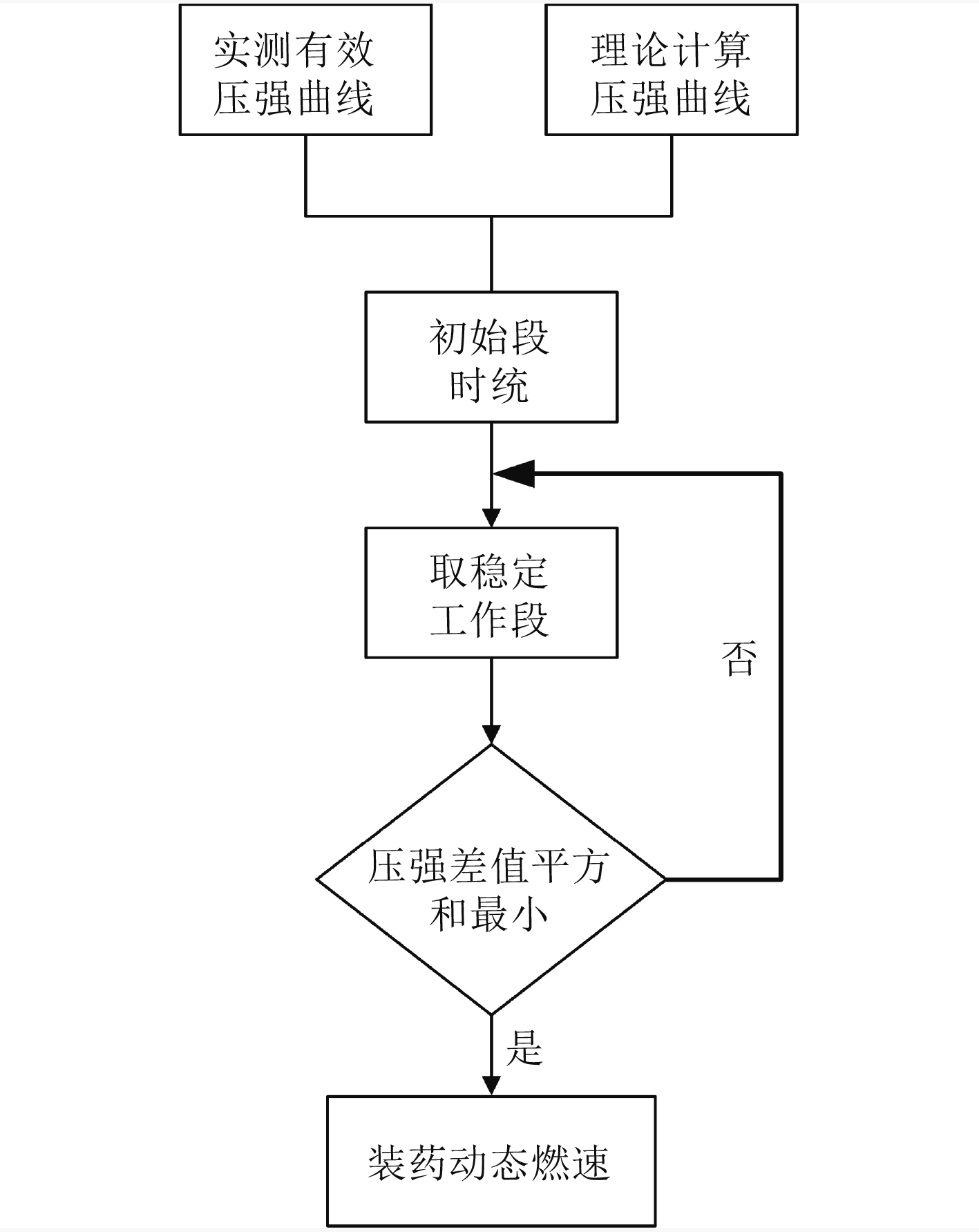图 3 动态燃速辨识流程图 Fig. 3 Flow chart of dynamic burning rate identification
4 动态燃速辨识结果表 1 动态燃速辨识结果 Tab.1 identification results of dynamic burning rate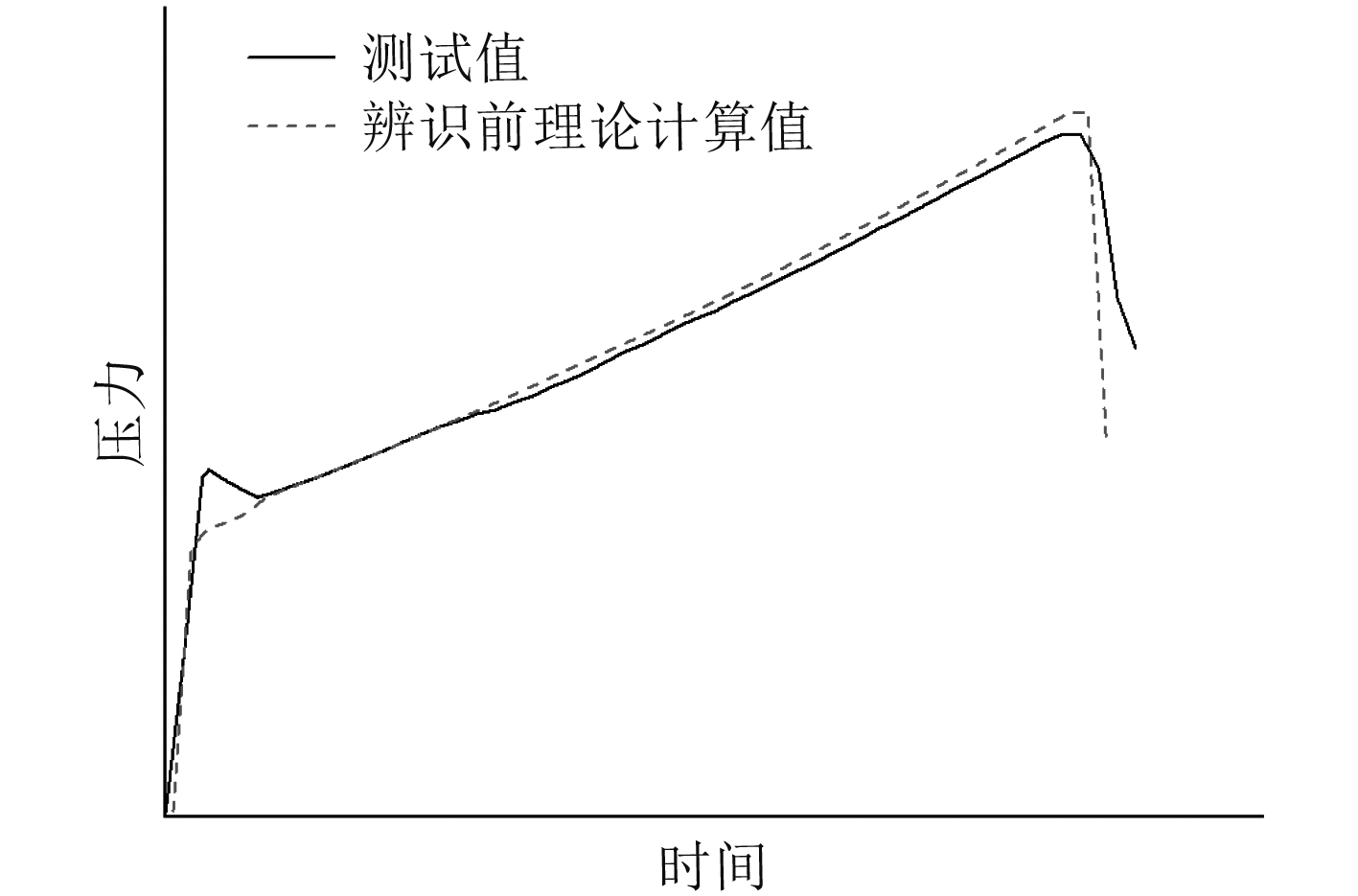图 4 静态燃速计算压强与测试压强曲线对比 Fig. 4 Comparison between calculated pressure and test pressure curve of static burning rate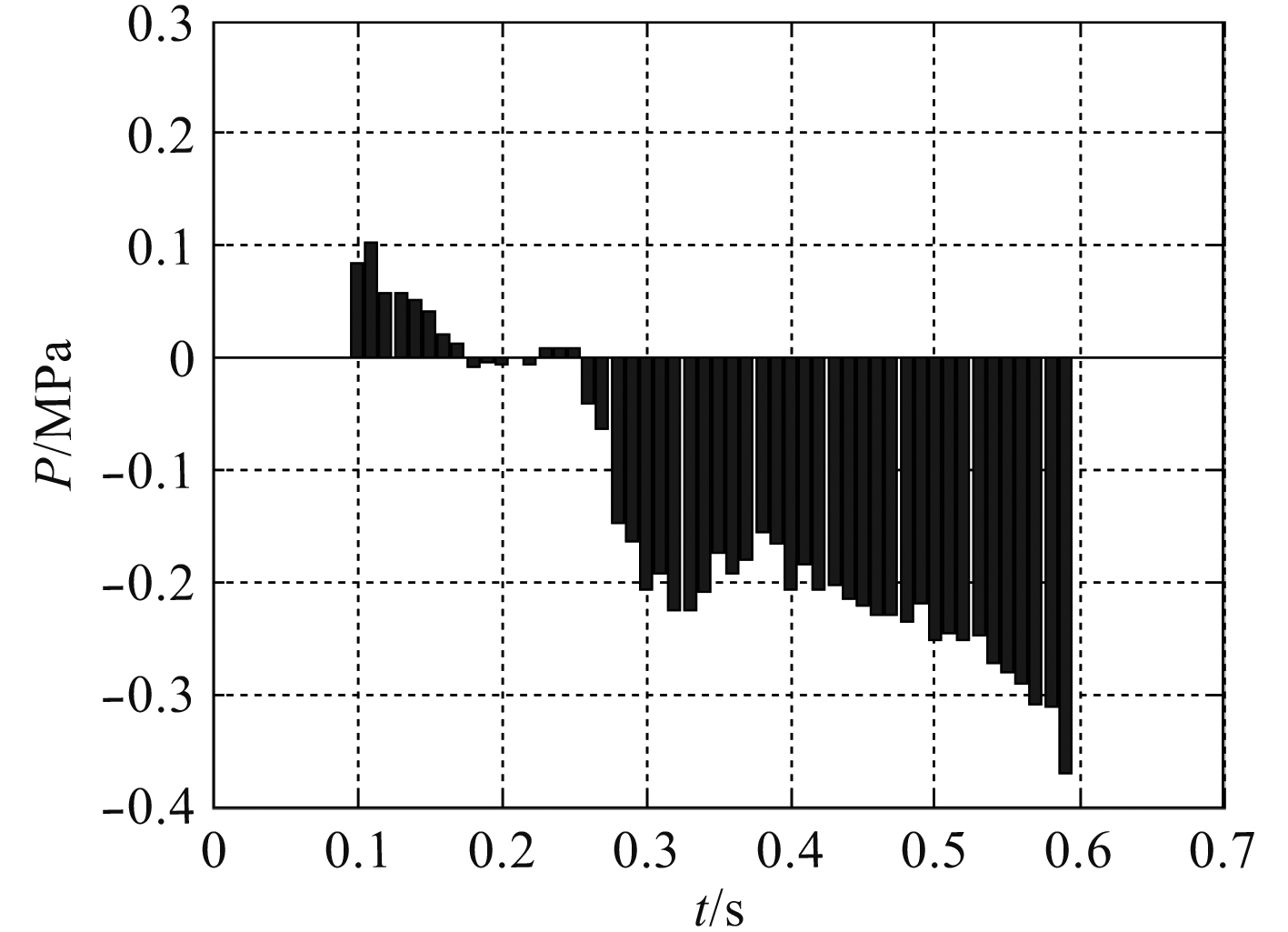图 5 辨识前压强偏差分布直方图 Fig. 5 Histogram of pressure deviation distribution before identification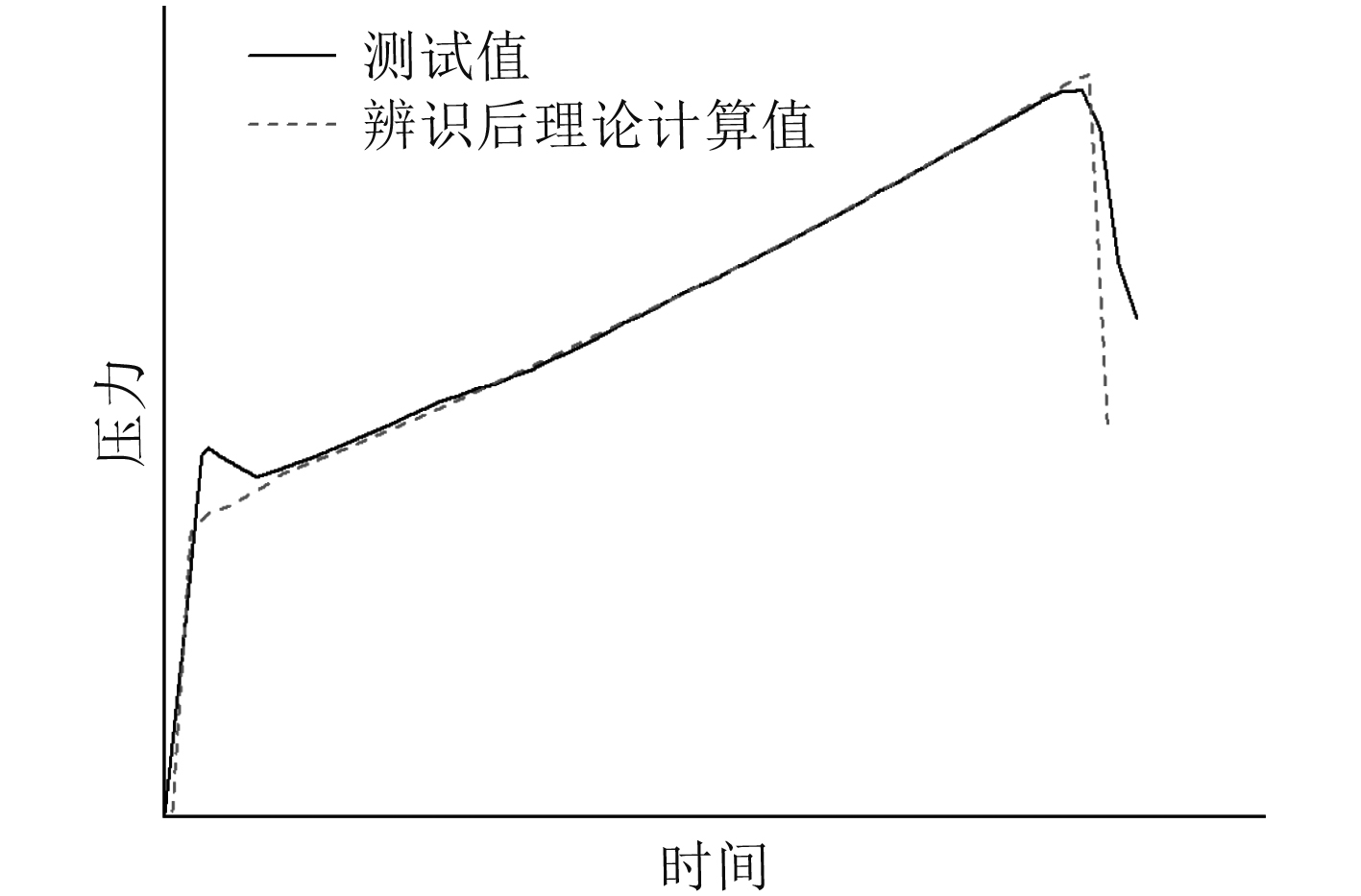图 6 动态燃速计算压强与测试压强曲线对比 Fig. 6 Comparison between calculated pressure and measured pressure curve of dynamic burning rate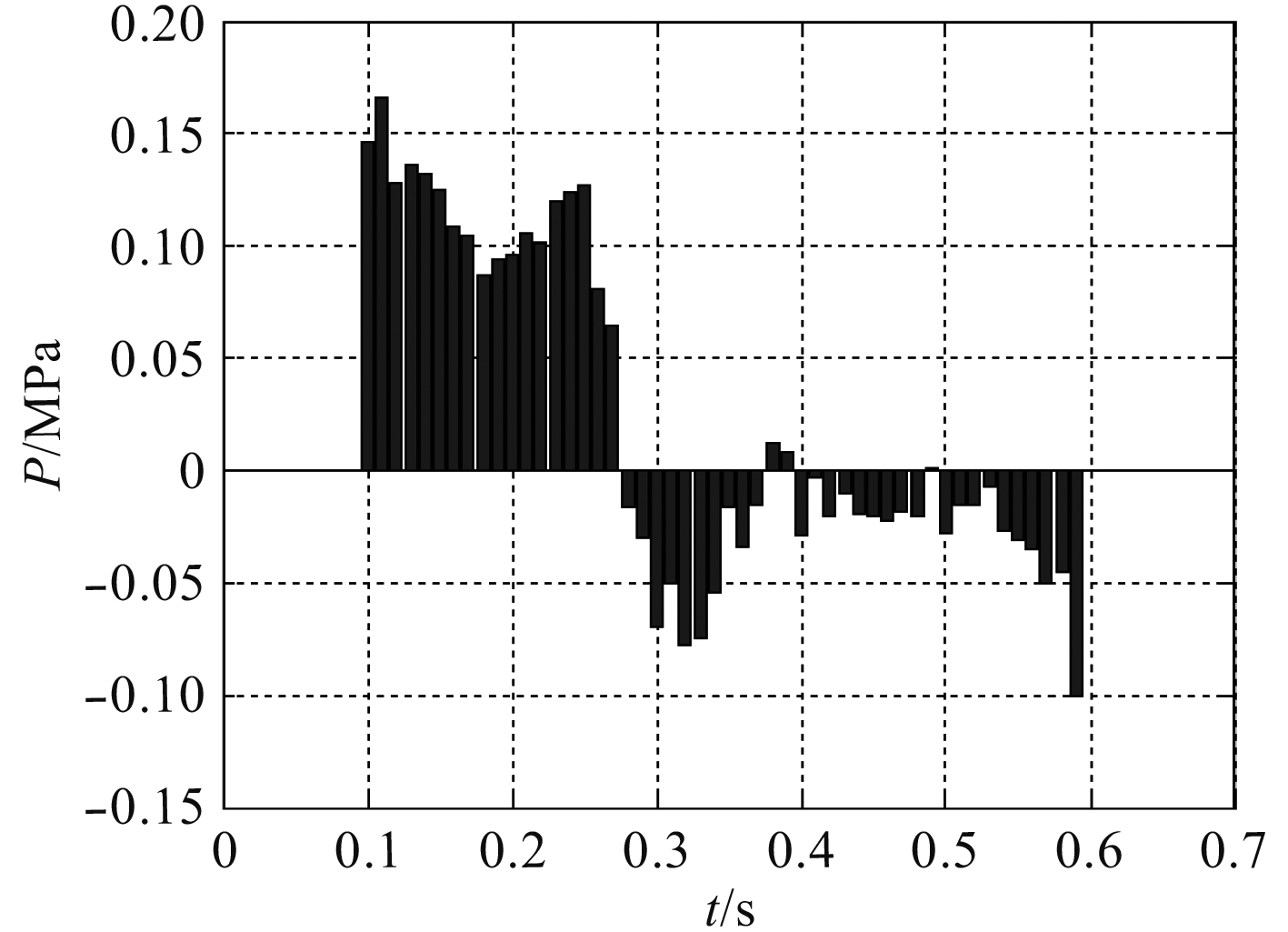图 7 辨识后压强偏差分布直方图 Fig. 7 Histogram of pressure deviation distribution after identification
5 结　语

1）改性双基推进剂出厂的 $\phi$ 5药条燃速为静态燃速，由于其取样方法与实际发动机工作过程存在差异，直接用于固体火箭发动机压强计算会产生偏差；

2）基于最小二乘法辨识出的固体火箭发动机装药燃速为动态燃速，利用该燃速可有效提高理论计算模型的预示精度；

3）根据多批装药动态燃速辨识结果可知，本文提出的动态燃速辨识方法具有较强的准确性和工程应用价值。

  李葆首. 固体推进剂性能[M]. 西安: 西北工业大学出版社. 1990, 12.  中国国家军用标准GJB770B-2005: 火炸药实验方法: 燃速测试方法706.1靶线法和706.2水下声发射法[S].  裴庆, 赵凤起, 等. 固体推进剂燃速测试技术研究进展[J]. 火炸药学报, 2015, 38(3): 9-14. PEI Qing, ZHAO Fengqi, et al. Research progress in the burning rate measurement technology of solid propellant[J]. Chinese Journal of Explosives & Propellants, 2015, 38(3): 9-14.  武晓松, 陈军, 等. 固体火箭发动机原理[M]. 北京: 兵器工业出版社, 2011.  马凯歌. 固体推进剂准动态燃速测试方法研究[D]. 西安: 西安电子科技大学, 2012. MA Kaige. Research on Test Method for Quasi-dynamic Burning Rate of Solid Propellant[D], Xi An: Xi Dian University, 2012.  唐金兰, 刘佩进. 固体火箭发动机原理[M]. 北京: 国防工业出版社, 2013.  李咸海, 王俊杰. 潜地导弹发射动力系统[M]. 哈尔滨: 哈尔滨工程大学出版社, 2000.  贾小勇, 徐传胜, 白欣. 最小二乘法的创立及其思想方法[J]. 西北大学学报(自然科学版), 2006(3): 507-511. JIA Xiaoyong, XU Chuansheng, BAI Xin. The invention and way of thinking on least squares[J]. Journal of Northwest University (Natural Science Edition), 2006(3): 507-511. DOI:10.3321/j.issn:1000-274X.2006.03.040